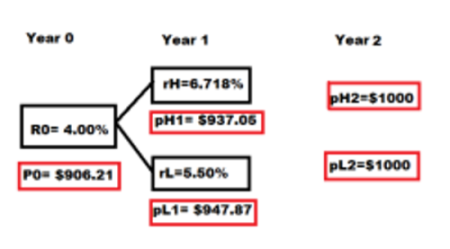# Using a 50%; 50% probabilities binary-interest-rate-tree model, and the following assumptions, ...

## Question:

Using a 50%; 50% probabilities binary-interest-rate-tree model, and the following assumptions,

 r0 = 4.000% rL = 5.500% rH = 6.718% volatility = 10%

Calculate the price of the 2-year 5.7% option-free bond.

## Binomial Tree:

Binomial Tree is one of the methods for valuation other being the Black Scholes Merton Model. It is a parsimonious model to value bonds, options, etc. Under this model, two possible values are determined and then discounted using the risk neutral probabilities and interest rate to determine the fair value.

Coupon Rate is 5.70% which means that if the face value of the bond is 1000, the coupon is 570. Time is 2 years. Volatility is 10% which is not required in this question. A pictoral representation of the interest rate tree and the prices is given below:Step1: First we calculate pH1 and pL1 at the end of year 1, when interest rates are 6.718% and 5.50% and prices at the end of year 2 are $1000 each. To calculate the prices at the end of year 1, we discount the future values with the respective interest rates as follows: {eq}pH1=\frac{pH2}{1+rH} and pL1= \frac{pL2}{1+rL} {/eq} So, we get: {eq}pH1=\frac{1000}{1.06178}=$937.05 {/eq}

And,

{eq}pL1=\frac{1000}{1.055}= $947.87 {/eq} Step 2: Now, we have a probability of 0.5 and 0.5 on each arm. So we take the average of the prices pH1 and pL1 to get$942.46. This price is standing at the end of year 1.

Step 3: To calculate today's price P0, we discount the average price with the rate 4.00% as shown below:

{eq}P0=\frac{942.46}{1.04}= $906.21 {/eq} Hence, today's price of the bond is calculated to be$906.21.